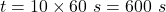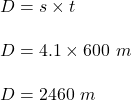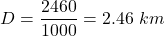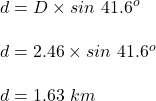## A kayak on a lake is moving at a constant speed of 4.1 m/s in a direction 41.6° north of west. After 10 min, find the following.(a) how far

Question

A kayak on a lake is moving at a constant speed of 4.1 m/s in a direction 41.6° north of west. After 10 min, find the following.(a) how far west the kayak has traveled km (b) how far north the kayak has traveled km

in progress 0
6 months 2021-07-29T20:13:00+00:00 1 Answers 5 views 0

1. Given :

A kayak on a lake is moving at a constant speed of 4.1 m/s in a direction 41.6° north of west.

To Find :

After 10 min :

(a) how far west the kayak has traveled km.

(b) how far north the kayak has traveled km.

Solution :

a) Time taken in seconds :.

Distance travelled in 600 s is :Distance in km is :b) Now , distance from the north is given by :Hence , this is the required solution .